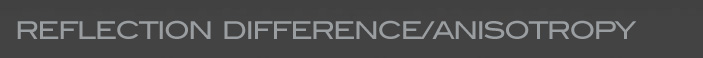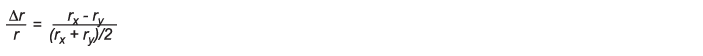Technology for Polarization Measurement# Reflection Difference/Anisotropy Spectroscopy

Reflectance Anisotropy Spectroscopy (RAS, also called RDS—Reflectance Difference Spectroscopy) is a normal incidence reflectance technique that utilizes the anisotropy of reconstructed semiconductor and metal surfaces and is capable of sensing the stoichiometry and symmetry of the uppermost atomic monolayers of cubic semiconductors and metals.

Complex reflectance anisotropy Δr/r due to the reduced symmetry at the surface of cubic crystals is measured. The signals are of the order of |Δr/r|<10−3 and carry information originating only from the surface region (the bulk beneath is optically isotropic and does not contribute to the measured spectrum).

### SETUPRAS measures the difference in the normal-incidence reflectances rx and ry for light that is linearly polarized parallel and perpendicular, respectively, to a chosen in-surface-plane axis x of a sample. Usually it is given as Δr/r (normalized to the average reflector, r).The RAS signal is determined by normalizing the ac components Iw and I2w to the respective dc component I0.Where δPEM is the retardation of the PEM, J1 and J2 are Bessel functions and ω is the frequency of the PEM.

### APPLICATIONS

• Growth Control in Production Lines
• "Watching" Atoms on Surfaces with Light
• Epitaxial Growth Monitoring
• Optical Surface Applications